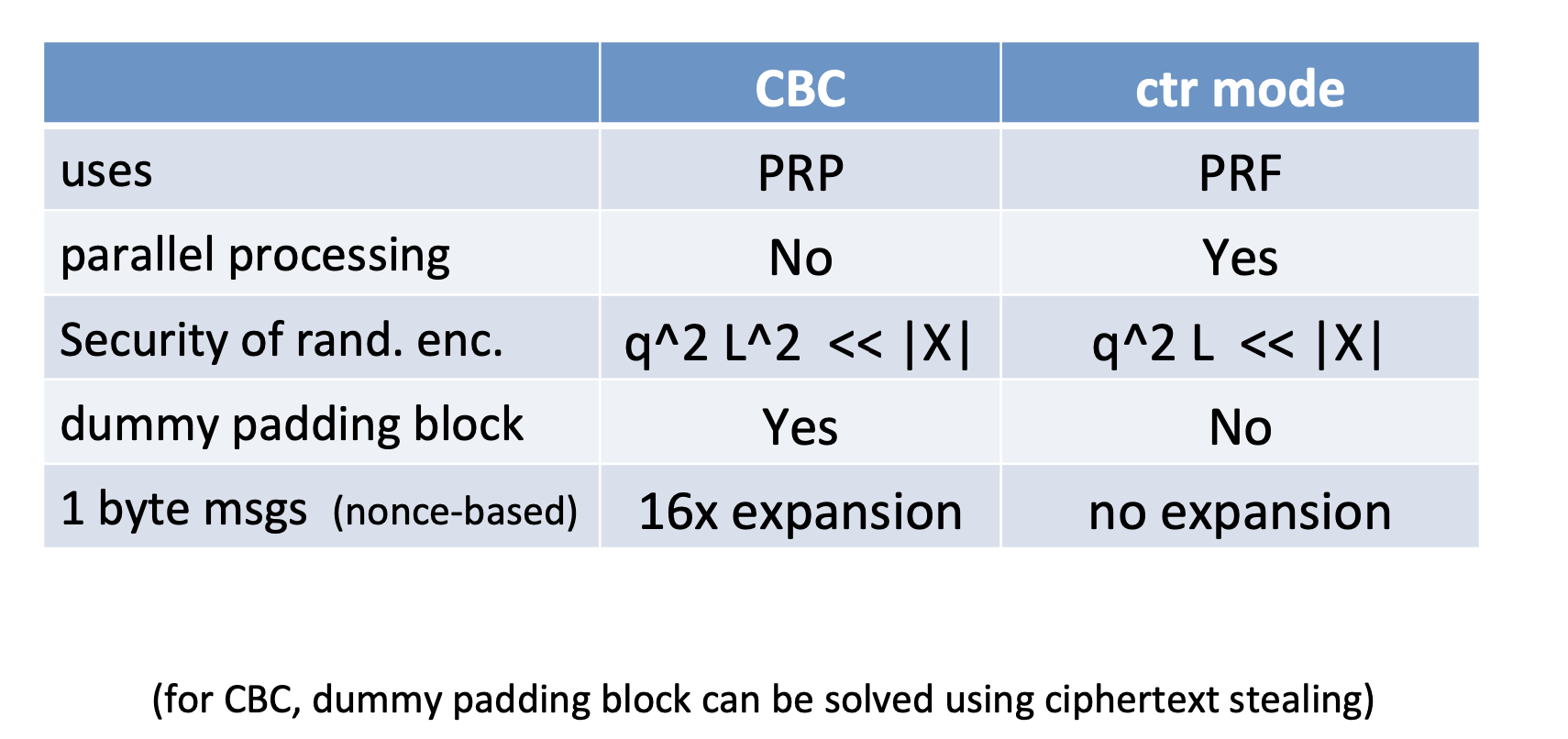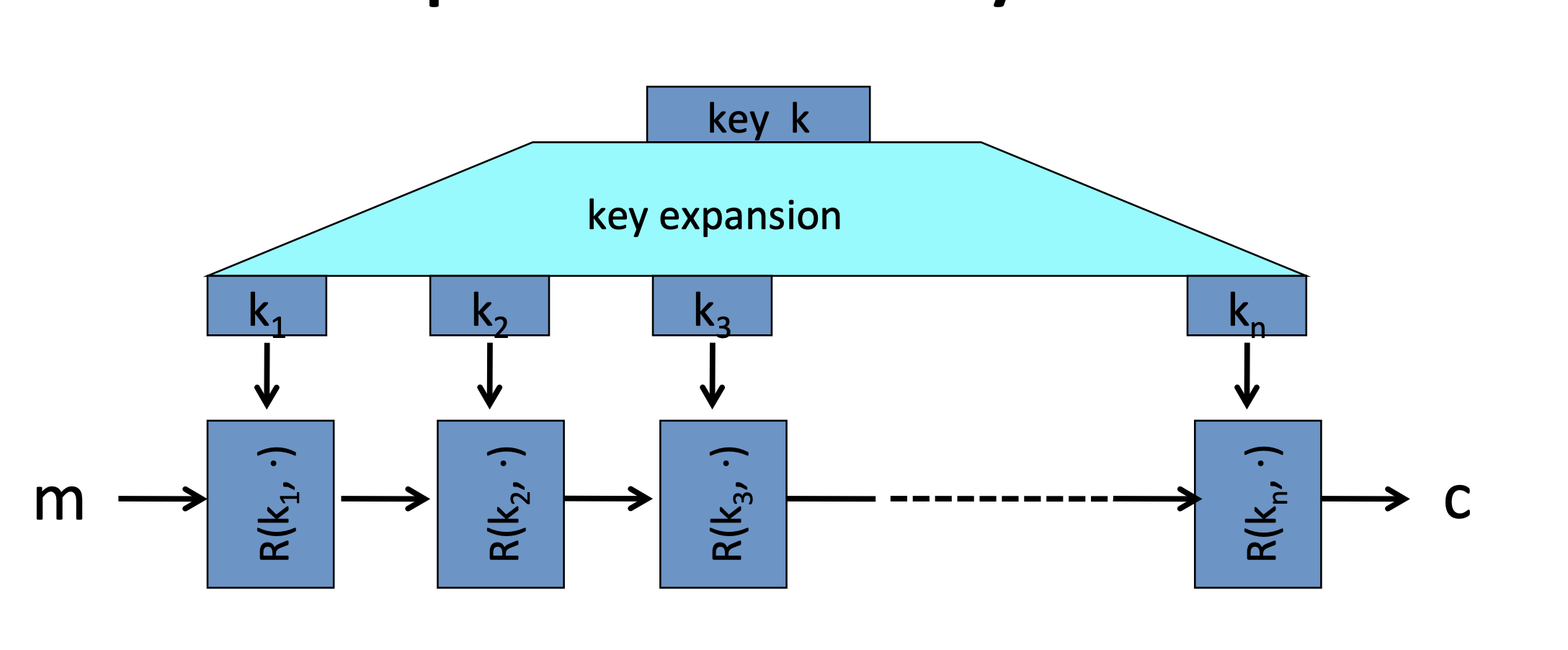# PRPs and PRFs

## PRF：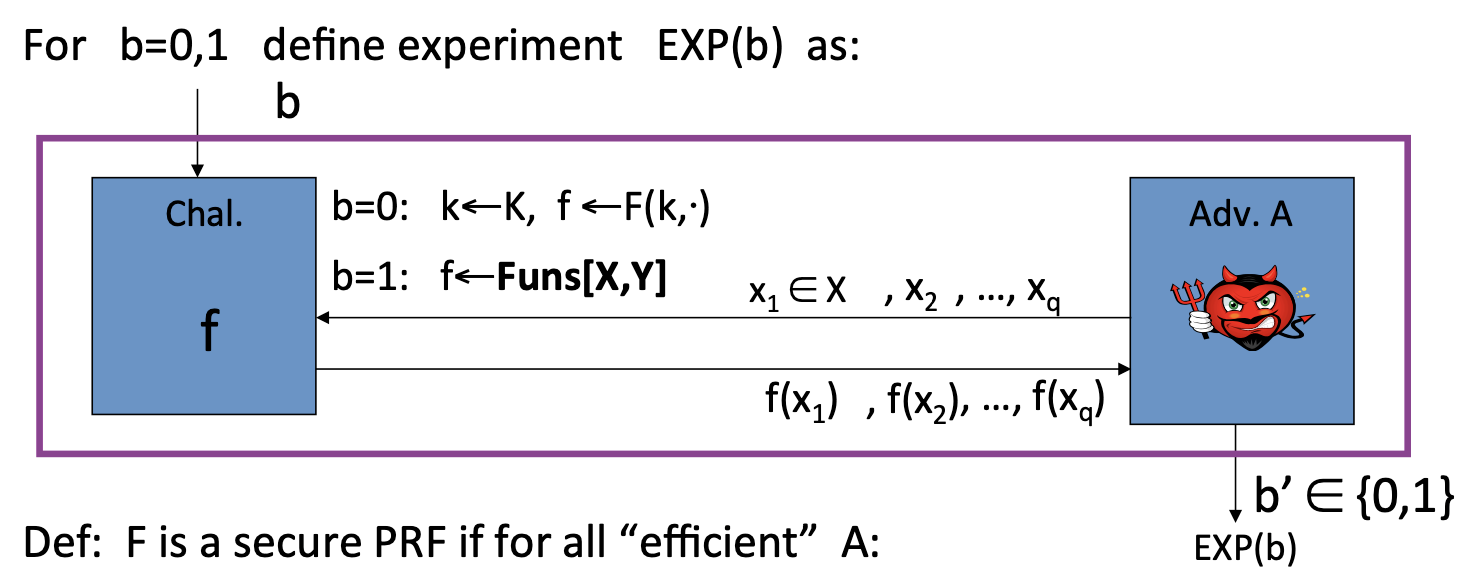Def(PRF): F is a secure PRF if for all “efficient” A:
$$\operatorname{Adv}_{\mathrm{PRF}}[\mathrm{A}, \mathrm{F}]:=\mid \operatorname{Pr}[\operatorname{EXP}(0)=1]-\operatorname{Pr}[\operatorname{EXP}(1)=1] \quad \text{is neg.}$$

## PRP: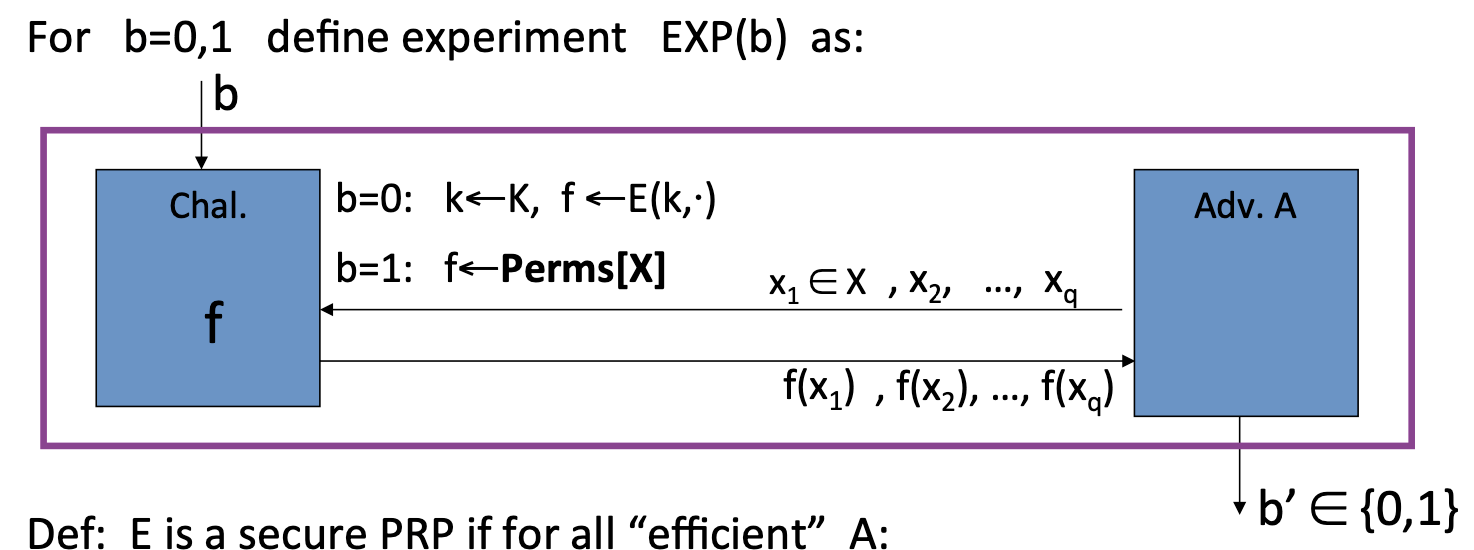Def(PRP): E is a secure PRP if for all “efficient” A:
$$\operatorname{Adv}_{\mathrm{PRP}}[\mathrm{A}, \mathrm{E}]:=\mid \operatorname{Pr}[\operatorname{EXP}(0)=1]-\operatorname{Pr}[\operatorname{EXP}(1)=1] \quad \text{is neg.}$$

Let X = {0,1}. Perms[X] contains two functions.

Consider the following PRP:

key space K={0,1}, PRP defined as:$\mathrm{E(k,x)=x\oplus k}$

Is this a secure PRP?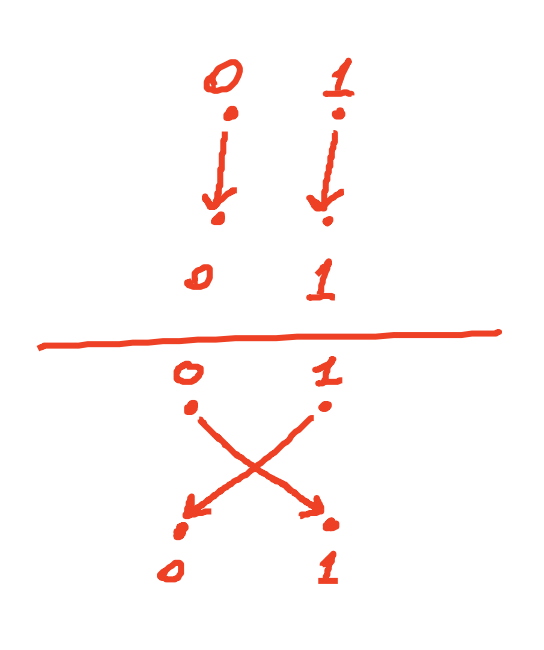## PRF Switching Lemma

Any Secure PRP is also a secure PRF, if |X| is sufficiently large.

【对于任意的安全PRP，当定义域 $X$ 足够大时，它也是安全PRF，所以AES也是安全的PRF。】

Lemma: Let E be a PRP over (K, X). Then for any q-query adversay A:
$$|\mathrm{Adv_{PRF}[A,E]-\mathrm{Adv_{PRP}[A, E]}}|<\mathrm{q^2/2|X|}$$

# One Time Key

one-time key: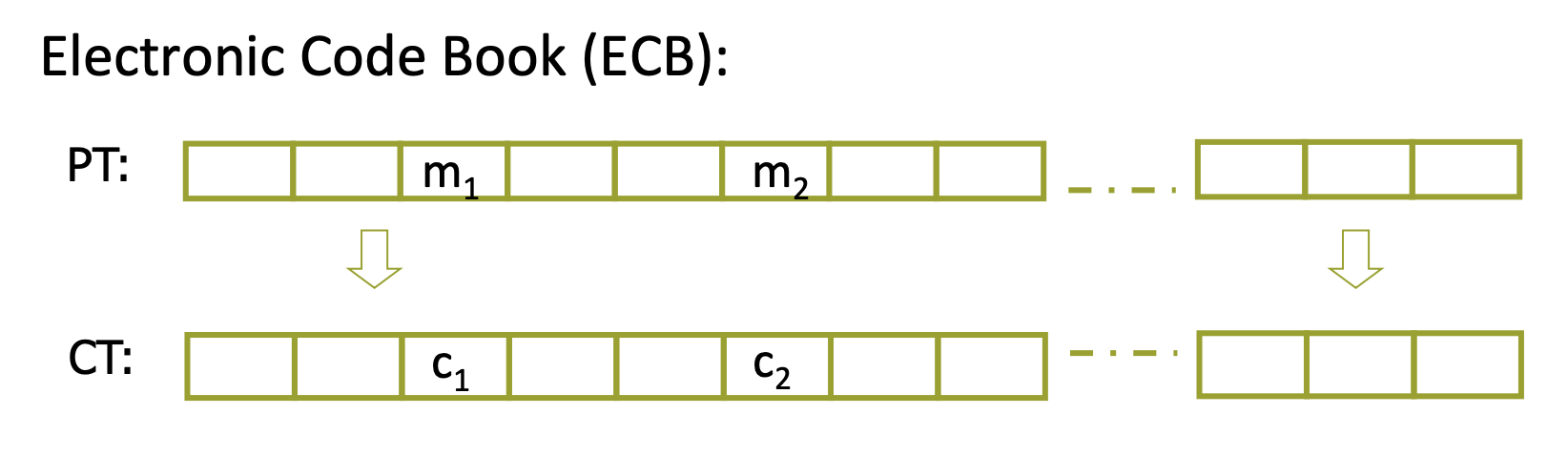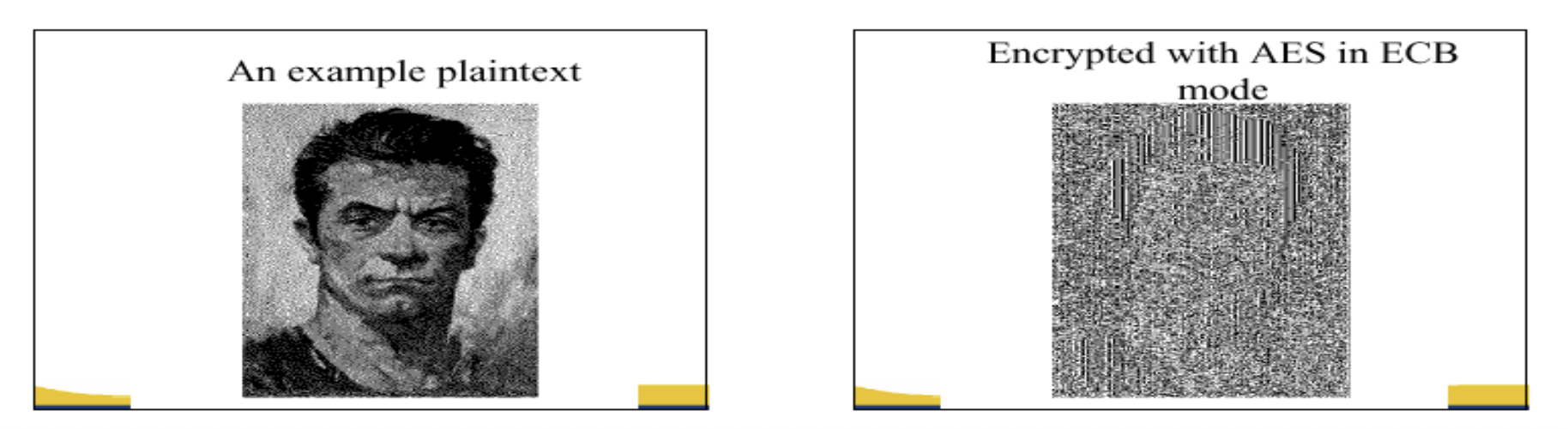## Semantic Security(one-time key)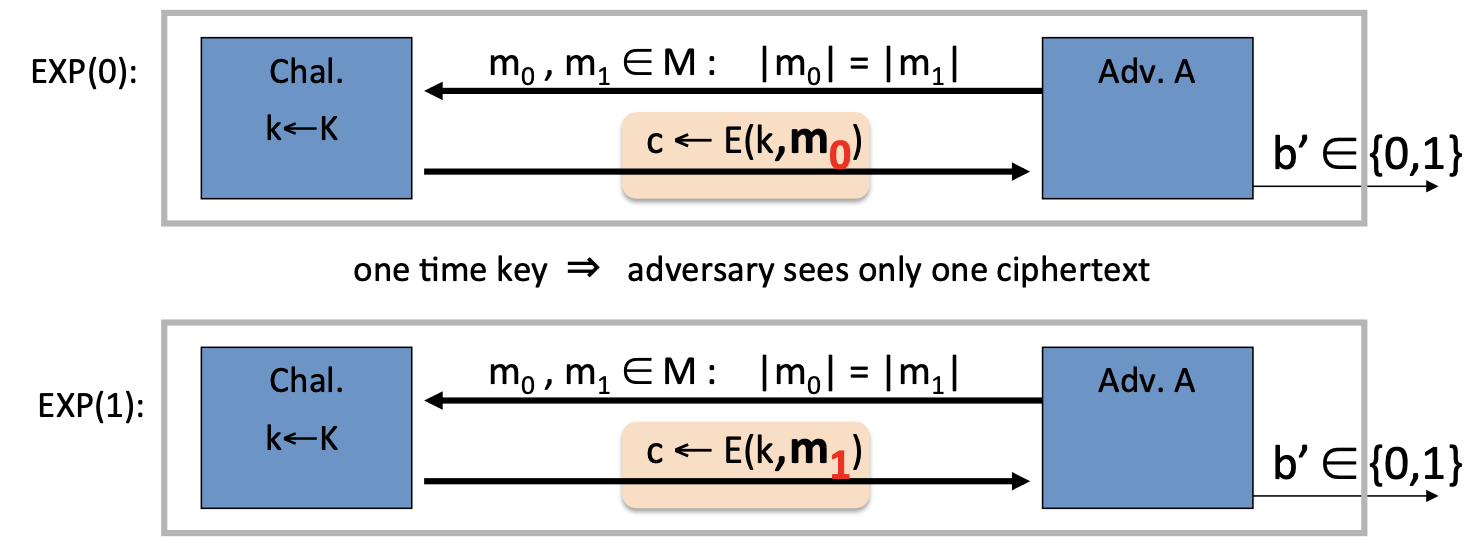$$\mathrm{Adv_{SS}[A,OTP]=|Pr[EXP(0)=1]-Pr[EXP(1)=1]} \quad \text{should be “neg”.}$$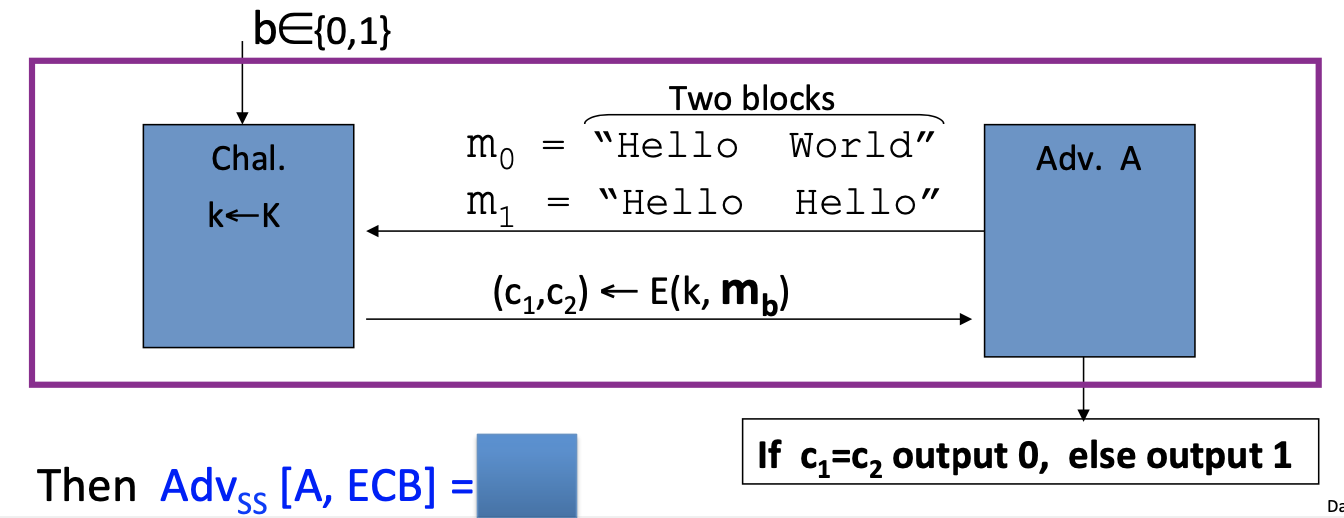## Det. CTR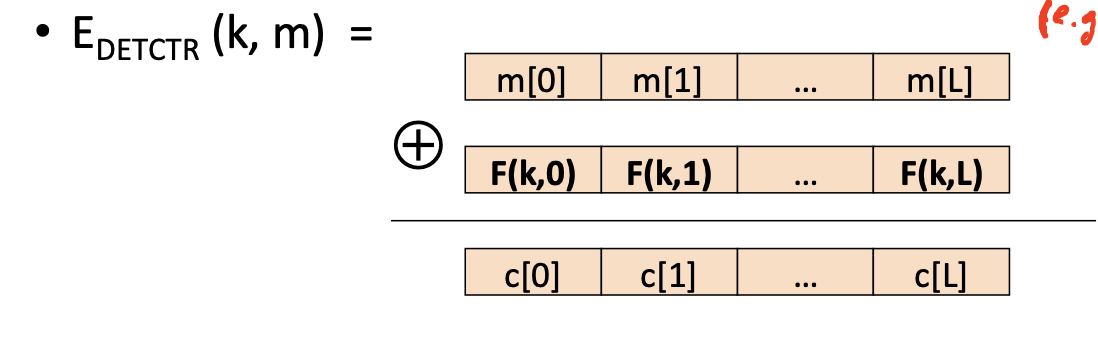Theorem:

For any L>0, if F is a secure PRF over (K,X,X) then $\mathrm{E_{DETCTR}}$ is sem. sec. cipher over $\mathrm{(K,X^L,X^L)}$ .

In particular, for any eff. adversary A attacking $\mathrm{E_{DETCTR}}$ there exists an eff. PRF adversary B s.t.:
$$\mathrm{Adv_{SS}[A,E_{DETCTR}]=2\cdot Adv_{PRF}[B,F]}$$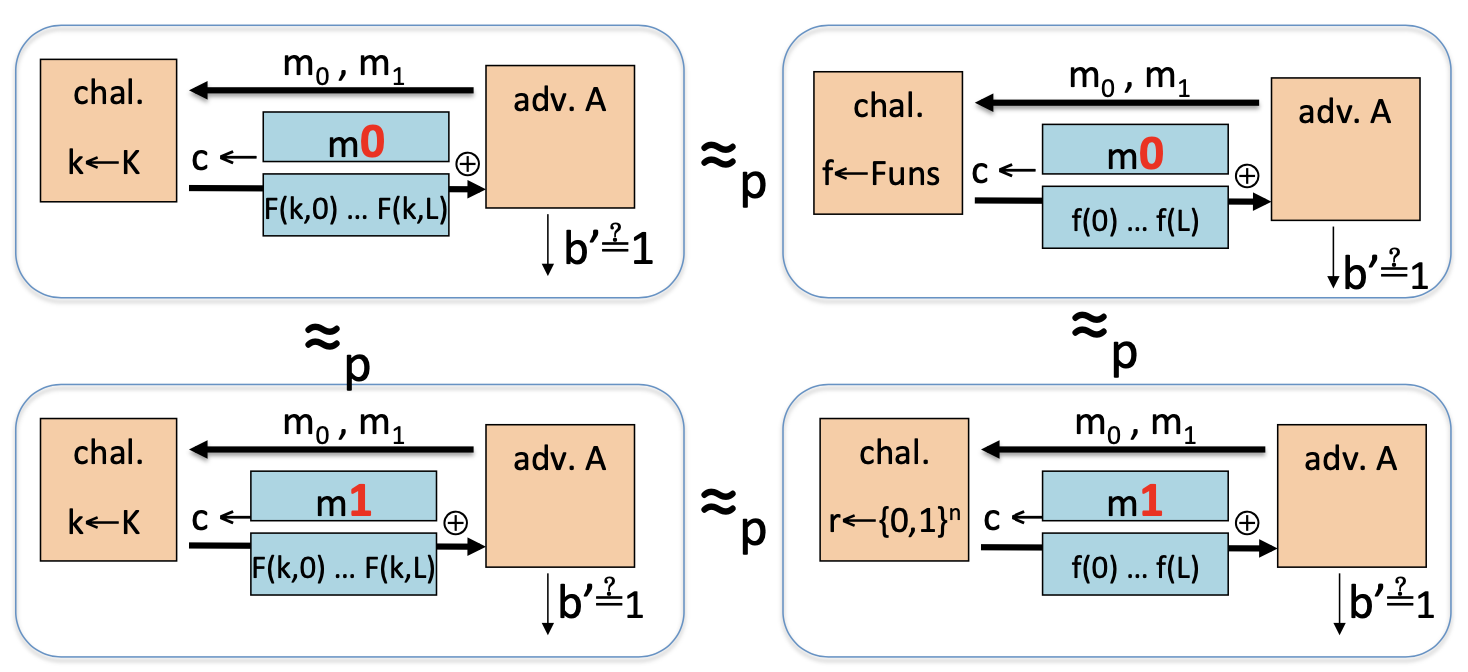# Many-time Key

many-time key的方式是说，同一条密钥用于加密多条消息，在这种情况下，攻击者有选择明文攻击的能力，可以获得他任意选择明文消息对应的密文。而攻击者的目标同样是破坏语义安全。

many-time key:

1. Adversary’s power: chosen-palintext attack(CPA): can obtain the encryption of arbitrary message of his choice.
2. Adversary’s goal: break semantic security.

## Semantic Security(many-time key)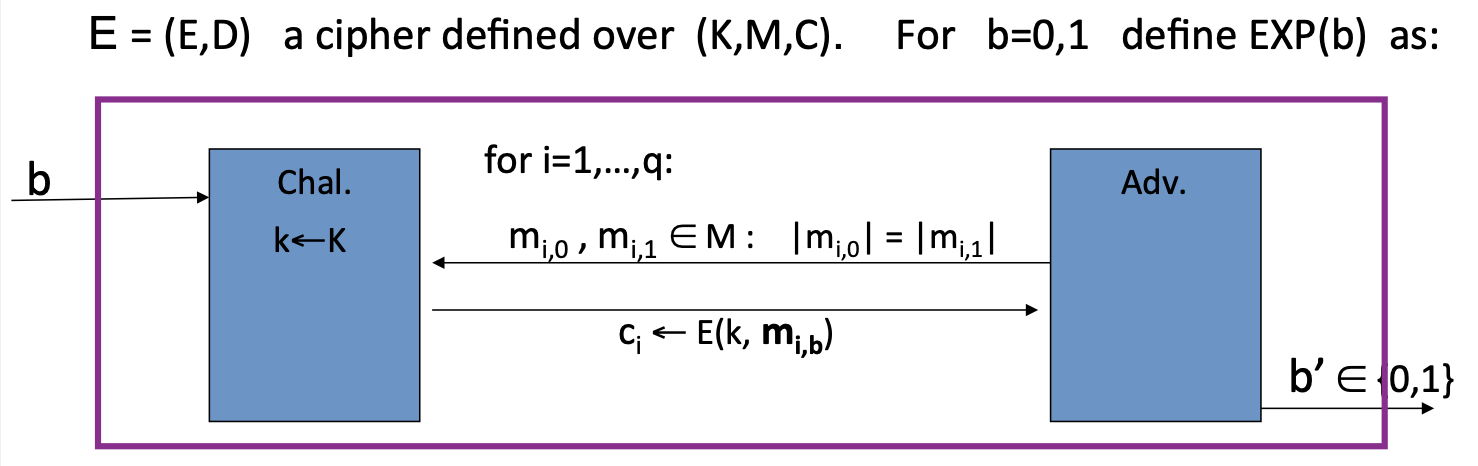Def: E is sem. sec. under CPA if for all “efficient” A:
$$\mathrm{Adv_{CPA}[A,E]=|Pr[EXP(0)=1]-EXP(1)=1|}\quad \text{is neg.}$$

## CPA Security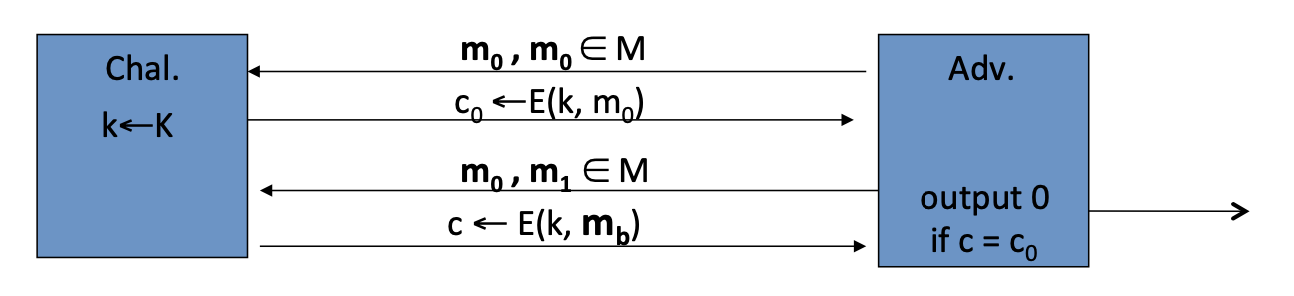### Solution 1: randomized encryption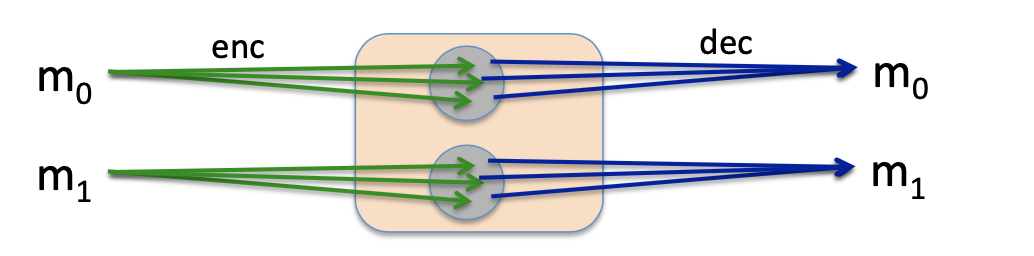### Solution 2: nonce-based Encryption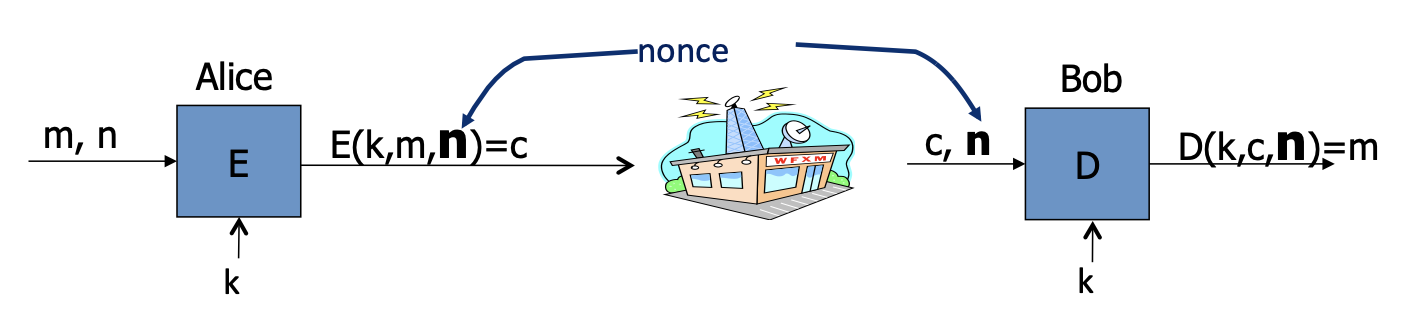nonce可以是一个计数器(counter)，也可以是一个随机值(random nonce)。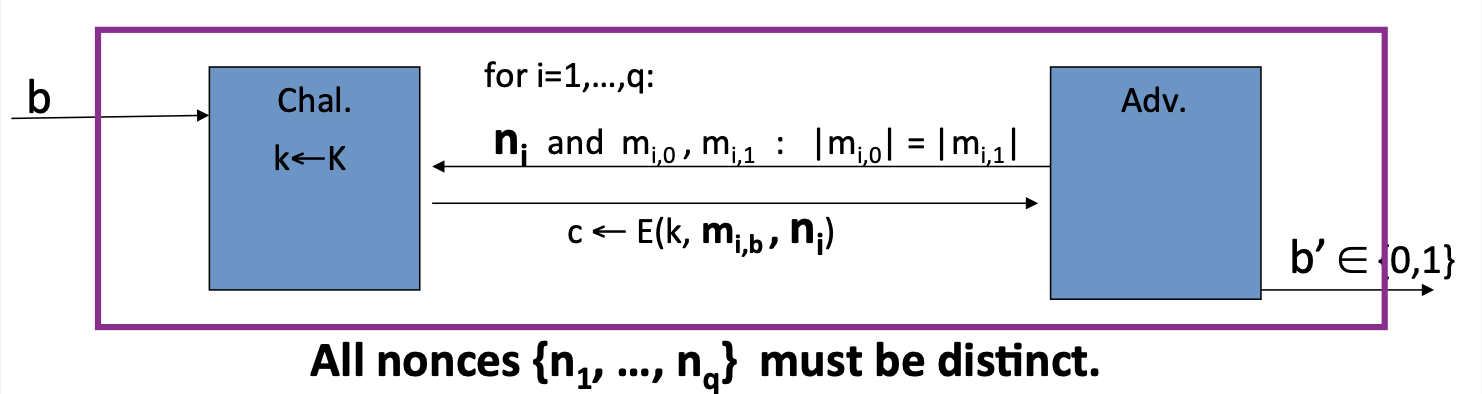Def : nonce-based E is sem. sec. under CPA if for all “efficient” A:
$$\mathrm{Adv_{nCPA}=|Pr[EXP(0)=1]-Pr[EXP(1)=1]|}\quad \text{is neg.}$$

## CBC

### CBC with random IV

Let $\mathrm{(E,D)}$ be a PRP.

$\mathrm{E_{CBC}(k,m)}$ : choose random $\mathrm{IV\in X}$ and do: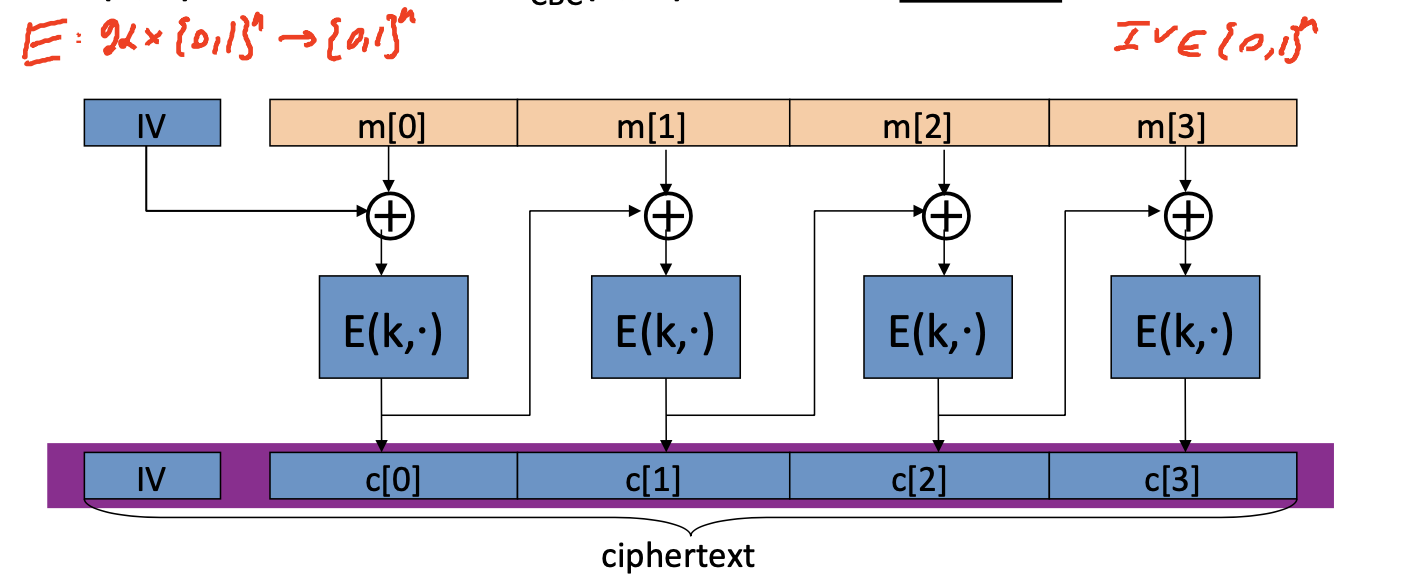IV是随机的，因此要把IV的值放在密文中一起发送给解密方。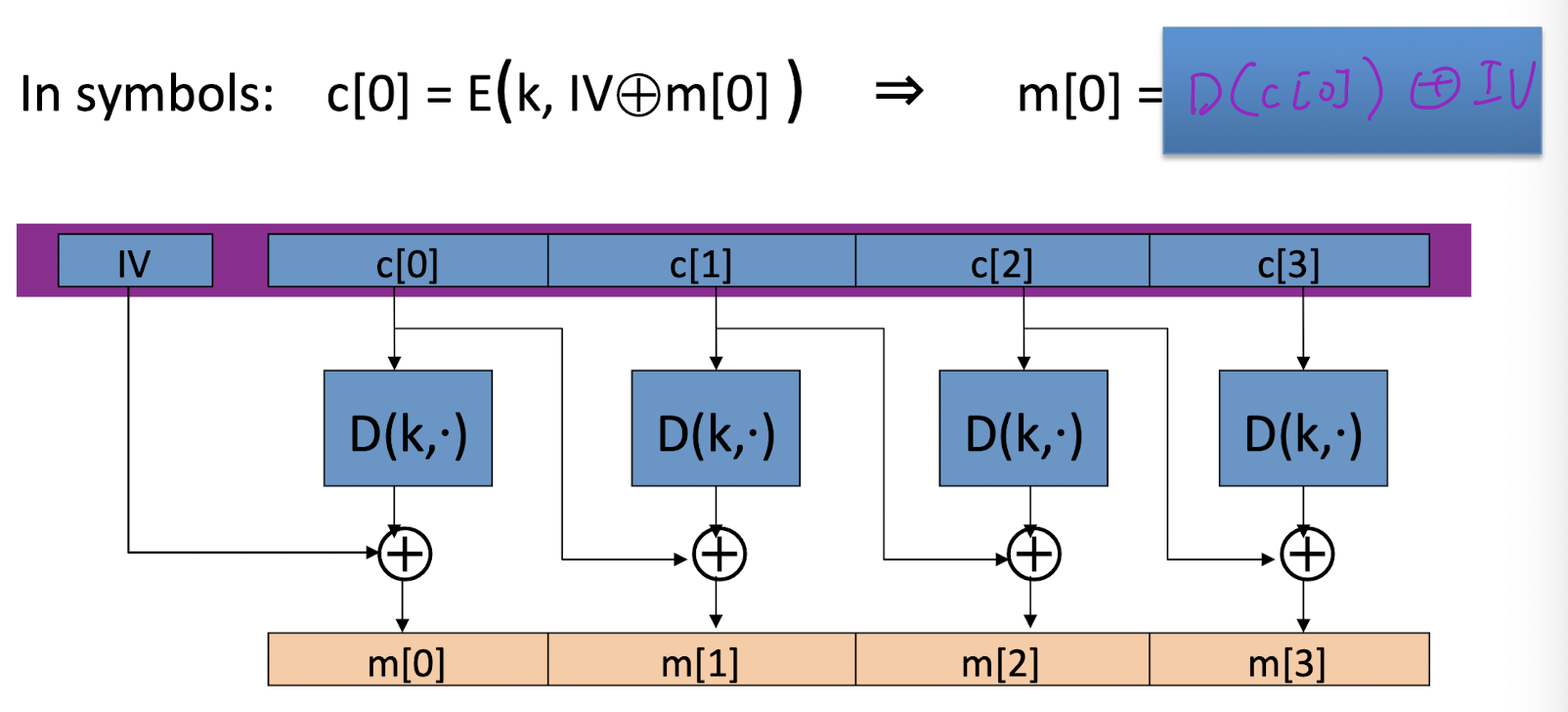### CBC: CPA Analysis

$$\mathrm{Adv_{CPA}[A,E_{CBC}]\le2\cdot Adv_{PRP}[B,E] +2q^2L^2/|X|}$$

CBC Theorem:

For any L>0, if E is a secure PRP over (K,X) then $\mathrm{E_{CBC}}$ is a sem. sec. under CPA over $\mathrm{(K,X^L,X^{L+1})}$ .

In particular, for a q-query adversary A attacking $\mathrm{E_{CBC}}$ , there exists a PRP adversary B s.t.:
$$\mathrm{Adv_{CPA}[A,E_{CBC}]\le2\cdot Adv_{PRP}[B,E] +2q^2L^2/|X|}$$
q = # messages encrypted with k, L = length of max message

PRP是安全的，$\mathrm{ Adv_{PRP}[B,E]}$ 是neg.，因此只有当 $\mathrm{q^2L^2<<|X|}$ 时，CBC才是安全的。

Example：

• 对于AES来说，$\mathrm{|X|=2^{128}}$ ，所以 $\mathrm{qL\lt 2^{48}}$ 。

因此，在 $2^{48}$ 个AES块后，必须改变密钥。

• 而对于DES来说，$\mathrm{|X|=2^{64}}$ ，所以 $\mathrm{qL\lt 2^{16}}$ 。

#### Warning: an attack on CBC with random IV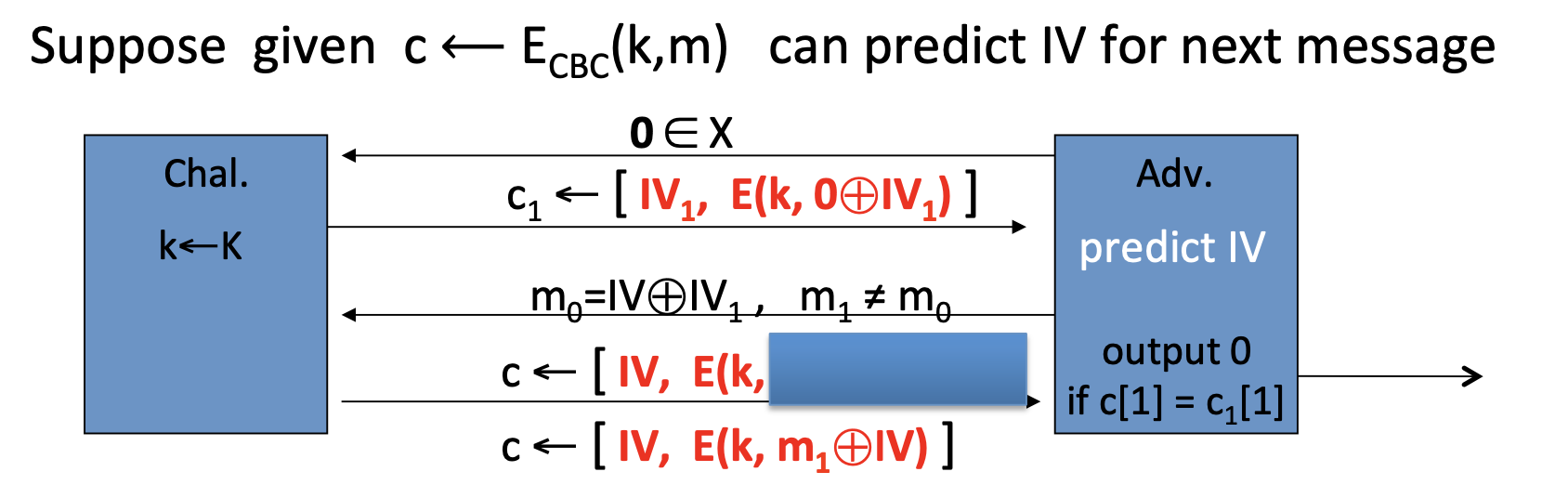CPA attack:

• 第一次询问
• 攻击者发送两条消息 $\mathrm{m_0=m_1=0}\in \mathrm{X}$
• 挑战者用 $\mathrm{c_1=[IV_1, E(k,0\oplus IV_1)]}$ 响应
• 攻击者通过密文预测下一条消息的IV
• 第二次询问
• 攻击者发送两条消息 $\mathrm{m_0=IV\oplus IV_1,m_1\ne m_0}$
• 挑战者的密文 $\mathrm{c=[IV,E(k,IV_1)]\quad or \quad c=[IV,E(k, m_1\oplus IV)]}$
• 攻击者的统计算法 A: output 0 if $\mathrm{c=c_1}$

### nonce-based CBC

CBC的另一种使用方式是基于unique nonce。

unique nonce的含义是，nonce可以不是随机的，但对于每一条消息，(key, nonce)对都必须是不同的。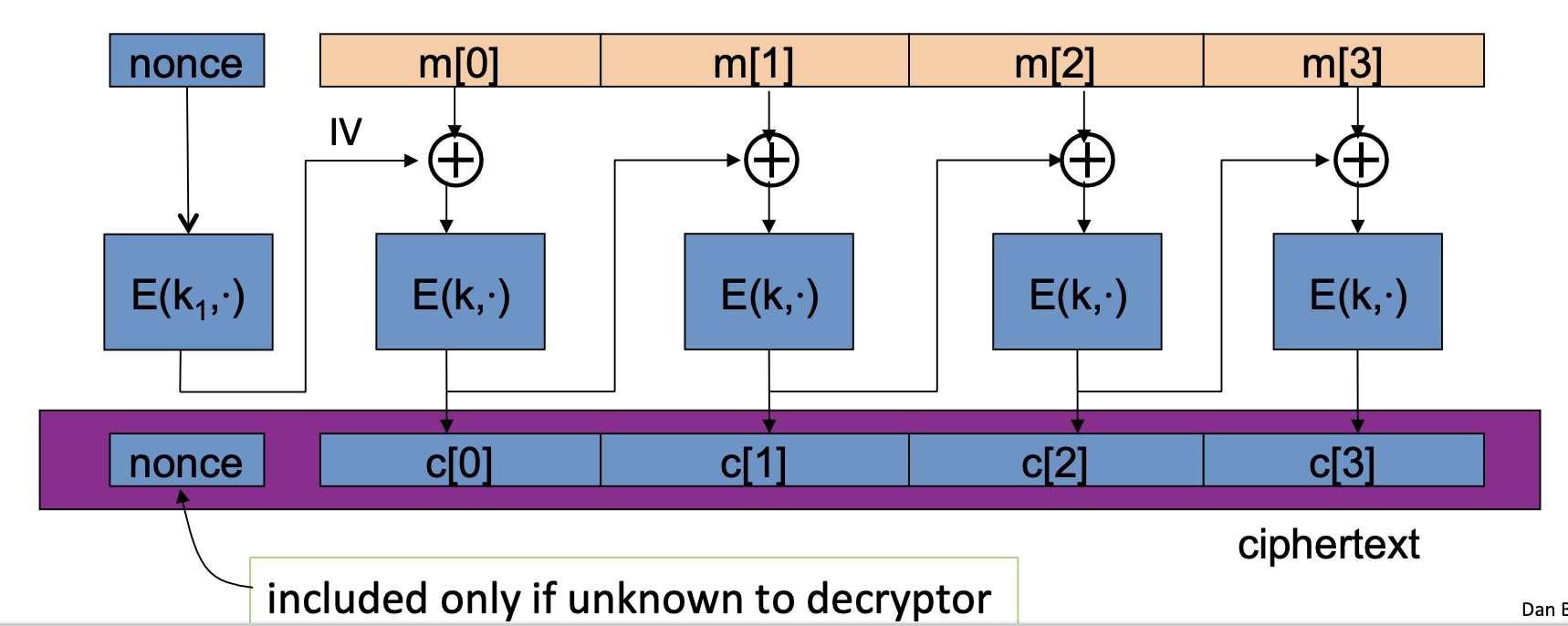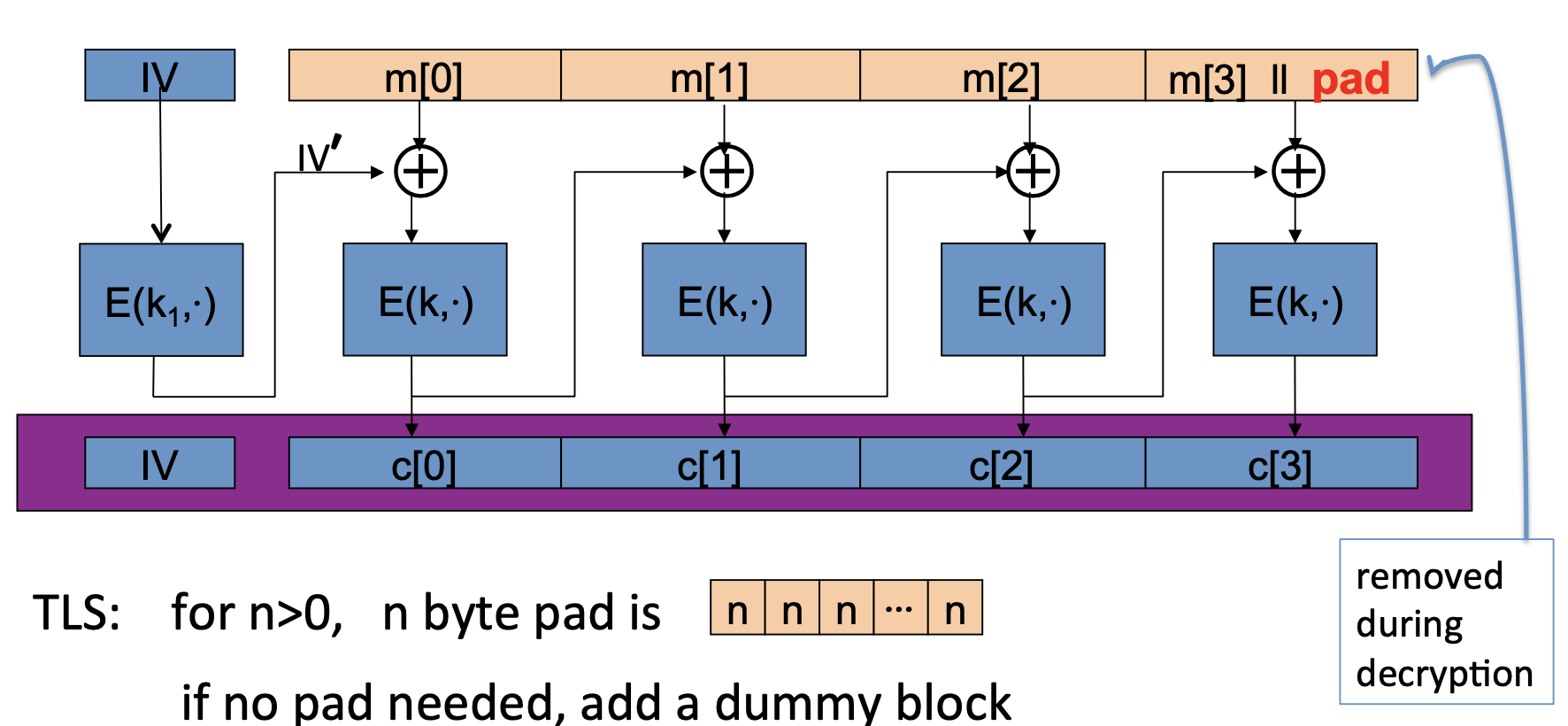## CTR

### rand ctr-mode

Let F be a secure PRF.

$\mathrm{E_{CBC}(k,m)}$ : choose random $\mathrm{IV\in X}$ and do: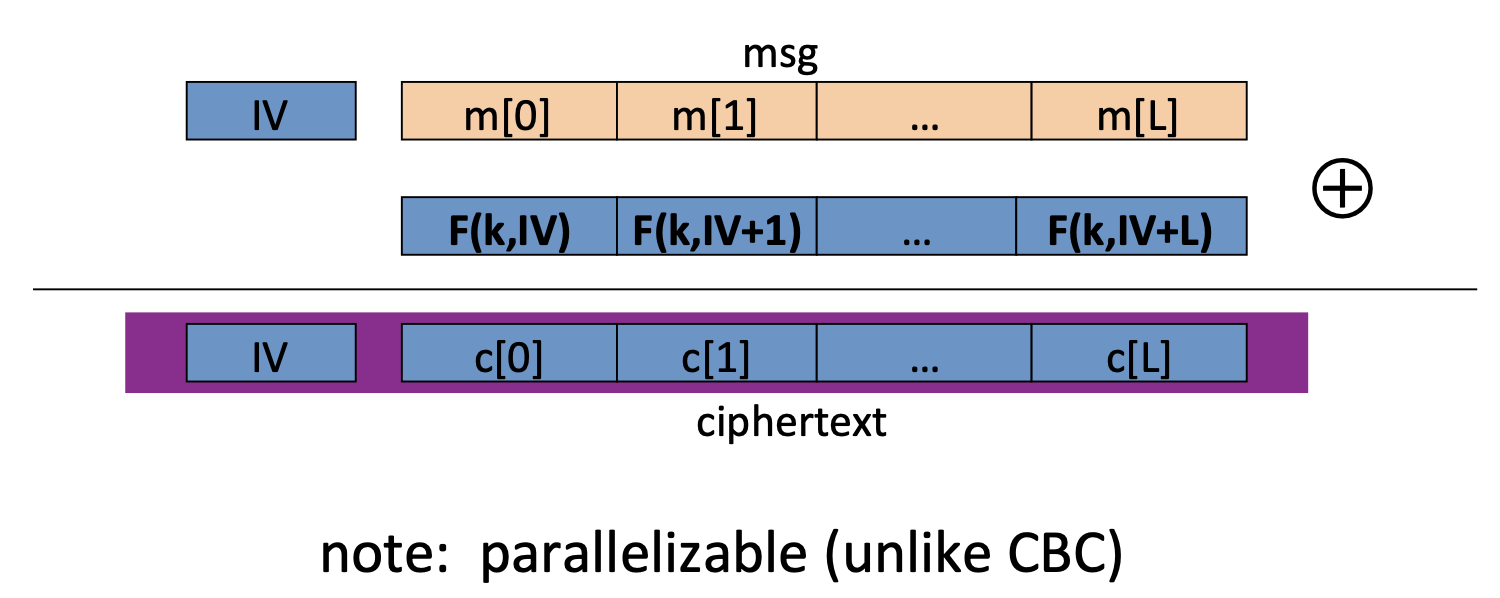CTR模式的最大优点是可以并行计算。

### nonce ctr-mode### CTR: CPA analysis

CTR Theorem:

For any L>0, if F is a secure PRF over (K, X, X) then $\mathrm{E_{CTR}}$ is a sem. sec. under CPA over $\mathrm{(K,X^L,X^{L+1})}$ .

In particular, for a q-query adversary A attacking $\mathrm{E_{CTR}}$ , there exists a PRF adversary B s.t.:
$$\mathrm{Adv_{CPA}[A,E_{CTR}]\le2\cdot Adv_{PRF}[B,F] +2q^2L/|X|}$$
q = # messages encrypted with k, L = length of max message

CTR-mode中，只有当 $\mathrm{q^2L}<<|X|$ ，才具备CPA-secure，比CBC好。

Example：

• 对于AES来说，$\mathrm{|X|=2^{128}}$ ，所以 $\mathrm{qL^{1/2}\lt 2^{48}}$ 。

因此，在 $2^{32}$ 条消息后（每条消息有 $2^{32}$个 AES块），必须改变密钥。

## CTR v.s. CBC